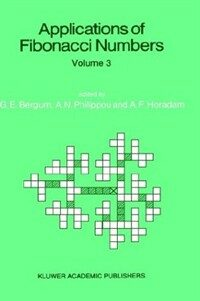> 상세정보

# 상세정보## Applications of Fibonacci numbers. v.3, : proceedings of 'The Third International Conference on Fibonacci Numbers and Their Applications', Pisa, Italy, July 25-29, 1988

자료유형
단행본
개인저자
Bergum, Gerald E. Philippou, Andreas N. Horadam, A. F.
서명 / 저자사항
Applications of Fibonacci numbers. v.3,. proceedings of 'The Third International Conference on Fibonacci Numbers and Their Applications', Pisa, Italy, July 25-29, 1988 / edited by G.E. Bergum, A.N. Philippou, and A.F. Horadam.
발행사항
Dordrecht ;   Boston :   Kluwer Academic Publishers,   c1990.
형태사항
xxiv, 357 p. : ill. ; 25 cm.
ISBN
079230523X (alk. paper)
일반주기
"Volume 3."
서지주기
Includes bibliographical references and index.
일반주제명
Fibonacci numbers --Congresses.
 000 01083camuuu200265 a 4500 001 000000903349 005 19990205140748.0 008 890920s1990 ne a b 10110 eng 010 ▼a 89024547 020 ▼a 079230523X (alk. paper) 040 ▼a DLC ▼c DLC ▼d DLC ▼d 244002 049 0 ▼l 452076654 ▼v 3 050 0 0 ▼a QA241 ▼b .I58 1988 082 0 0 ▼a 512/.72 ▼2 20 090 ▼a 512.72 ▼b B499a ▼c 3 100 1 ▼a Bergum, Gerald E. 245 1 0 ▼a Applications of Fibonacci numbers. ▼n v.3,. ▼p proceedings of 'The Third International Conference on Fibonacci Numbers and Their Applications', Pisa, Italy, July 25-29, 1988 / ▼c edited by G.E. Bergum, A.N. Philippou, and A.F. Horadam. 260 ▼a Dordrecht ; ▼a Boston : ▼b Kluwer Academic Publishers, ▼c c1990. 300 ▼a xxiv, 357 p. : ▼b ill. ; ▼c 25 cm. 500 ▼a "Volume 3." 504 ▼a Includes bibliographical references and index. 650 0 ▼a Fibonacci numbers ▼x Congresses. 700 1 ▼a Philippou, Andreas N. 700 1 ▼a Horadam, A. F. 711 2 ▼a International Conference on Fibonacci Numbers and Their Applications ▼n (3rd : ▼d 1988 : ▼c Pisa, Italy)

### 소장정보

No. 소장처 청구기호 등록번호 도서상태 반납예정일 예약 서비스
No. 1 소장처 청구기호 512.72 B499a 3 등록번호 452076654 도서상태 대출가능 반납예정일 예약 서비스

### 컨텐츠정보

#### 목차

```The Role of the Fibonacci Sequence in the Isolation of the Real Roots of Polynomial Equations.- A GCD Property on Pascal's Pyramid and the Corresponding LCM Property of the Modified Pascal Pyramid.- Translatable and Rotatable Configurations Which Give Equal Product, Equal GCD and Equal LCM Properties Simultaneously.- Fibonacci Length of Generating Pairs in Groups.- A Generalization of Fibonacci Trees.- Generalized Fibonacci Numbers are Rounded Powers.- On Generalized Fibonacci Numbers of Graphs.- An Investigation of Sequences Derived from Hoggatt Sums and Hoggatt Triangles.- Representation of Natural Numbers as Sums of Fibonacci Numbers: An Application to Modern Cryptography.- A Note on Ramifications Concerning the Construction of Pythagorean Triples from Recursive Sequences.- On the Representation of {Fkn/Fn}, {Fkn/Ln
}, {Lkn/Ln} and {Lkn/Fn} as Zeckendorf Sums.- Functional Recurrences.- Concentric Cycles in Mosaic Graphs.- Fibonacci Triangles.- Mosaic Numbers of Fibonacci Trees.- Falling Factorial Polynomials of Generalized Fibonacci Type.- Some Notes on Fibonacci Binary Sequences.- Congruences for Weighted and Degenerate Stirling Numbers.- An Inverse Theorem on Fibonacci Numbers.- Some Results on Divisibility Sequences.- On Mental Calculation of Repeating Decimals, Finding Fibonacci Numbers and a Connection to Pascal's Triangle.- Diophantine Representation of Fibonacci Numbers Over Natural Numbers.- On Prime Divisors of the Terms of Second Order Linear Recurrence Sequences.- An Alternating Product Representation for Real Numbers.- Maximum Length of the Euclidean Algorithm and Continued Fractions in F(X).- Recurrence Relations in Sinusoids and Their Applications to Spectral Analysis and to the Resolution of Algebraic Equations.- A Recurrence Relation for Gaussian Multinomial Coefficients.- Some Binomial Fibonacci Identities.- A Survey of Properties of Third Order Pell Diagonal Functions.- Multivariate Fibonacci Polynomials of Order K and the Multiparameter Negative Binomial Distribution of the Same Order.- Longest Circular Runs with an Application in Reliability Via the Fibonacci-Type Polynomials of Order K.- Fibonacci Numbers and an Algorithm of Lemoine and Katai.- Generalizations of Sequences of Lucas and Bell.- Distribution of Residues of Certain Second-Order Linear Recurrences Modulo P.- The Fibonacci Tree, Hofstadter and the Golden String.- Three Number Trees - Their Growth Rules and Related Number Properties.```

김대수 (2022)

민만식 (2022)